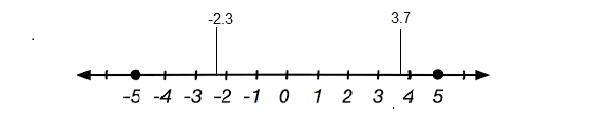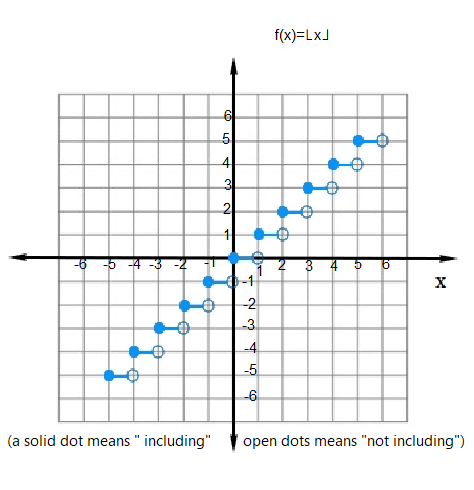June 28, 2022

# Greatest Integer Function

The Greatest integer function

is defined as   ,   where    denotes the greatest integer that is less than or equal to .

In set  notation we would write this as   . It is also called the floor function  and step function.

Example.  ,      and

#Domain and Range of Greatest integer function

Domain of greatest integer function is  ( the set of all real numbers) and range is ( the set of all integers ) as it only attains integer value.

## Graph of Greatest integer function

Let be the greatest  integer function define as

for all

Let us calculate some value of for different value of  .

The graph of greatest integer function is given below.### Greatest integer function is neither one-one nor onto

A function from to is said to be one-one if whenever then  . It means distinct elements have distinct image.

Here is given by  ,

we can see that      and .

but

Hence   is not one-one.

A function from to is called onto if for all there is an such that

Now consider .

It is known that     is always an integer.  Thus there does not exists any element  such that .  Thus  is not onto.

Hence  , the Greatest integer function is neither one-one nor onto.

#### Continuity  and differentiability of  greatest integer function

Continuity and differentiability are properties of a function at a specific point rather than properties of a function as a whole . A function is said to be continuous at a point c  if each of the following condition is satisfied.

(i) exists.  (c  is in the domain of )

(ii)  exists and

(iii)

Geometrically, this means that there is no gap, split  or missing point for at  and that a pencil could be moved along the graph of f(x)  through without lifting it off the graph.

Greatest integer function is continous at all points apart from integral values of . It is also differentiable with , at all points apart from integral values of .

Let be an integer .Then we can find a sufficiently small  real number such that   whereas . Thus

and .

Since  these limits cannot be equal  to each other for any , the function is discontinous at every integral points.

The greatest integer function is continuous at evety real no. other than integers.  For example

Let’s take x=1.5 . Then

In general,   if  is any  number which is not an integer and   is an integer , then    .#### Bina singh

View all posts by Bina singh →
IBPS Clerk Exam Date 2022 Out SSC MTS Admit Card 2022 Government Exam Calendar July 2022 CBSE Class 11 Mathematics Revised Syllabus 2022-2023 UGC NET 2022 Exam Date